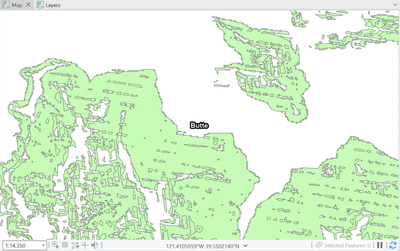# Why is my slope analysis causing a weird striping effect?

125
2
03-15-2023 09:17 AM
Labels (2)by
New Contributor III

I have a collection of elevation DEMs, I've projected them into the UTM zone I'm working in.

I use the following to process them, but it results in a strange striping effect:

• Slope (giving me a percentage)
• Reclass the raster into 30% and above
• Convert the raster to polygon

What might be causing this?

Here is some of the metadata from the DEMs:

• NED data sources have a variety of elevation units, horizontal datums, and map projections. In the
NED assembly process the elevation values are converted to a common coordinate reference
system and units. Decimal meters are the units for elevation values, NAD83 is the horizontal
datum, and all the data are recast in a geographic coordinate system.

Here is the result:Tags (5)
1 Solution

Accepted SolutionsbyEsri Regular Contributor

when you used ProjectRaster did you use ResamplingTechnique = Bilinear?

from Slope's help

• When the input raster needs to be resampled, the bilinear technique will be used. An example of when an input raster may be resampled is when the output coordinate system, extent, or cell size is different from that of the input.

2 RepliesbyEsri Regular Contributor

when you used ProjectRaster did you use ResamplingTechnique = Bilinear?

from Slope's help

• When the input raster needs to be resampled, the bilinear technique will be used. An example of when an input raster may be resampled is when the output coordinate system, extent, or cell size is different from that of the input.by
New Contributor III

Thank you Steve, this seems to work!